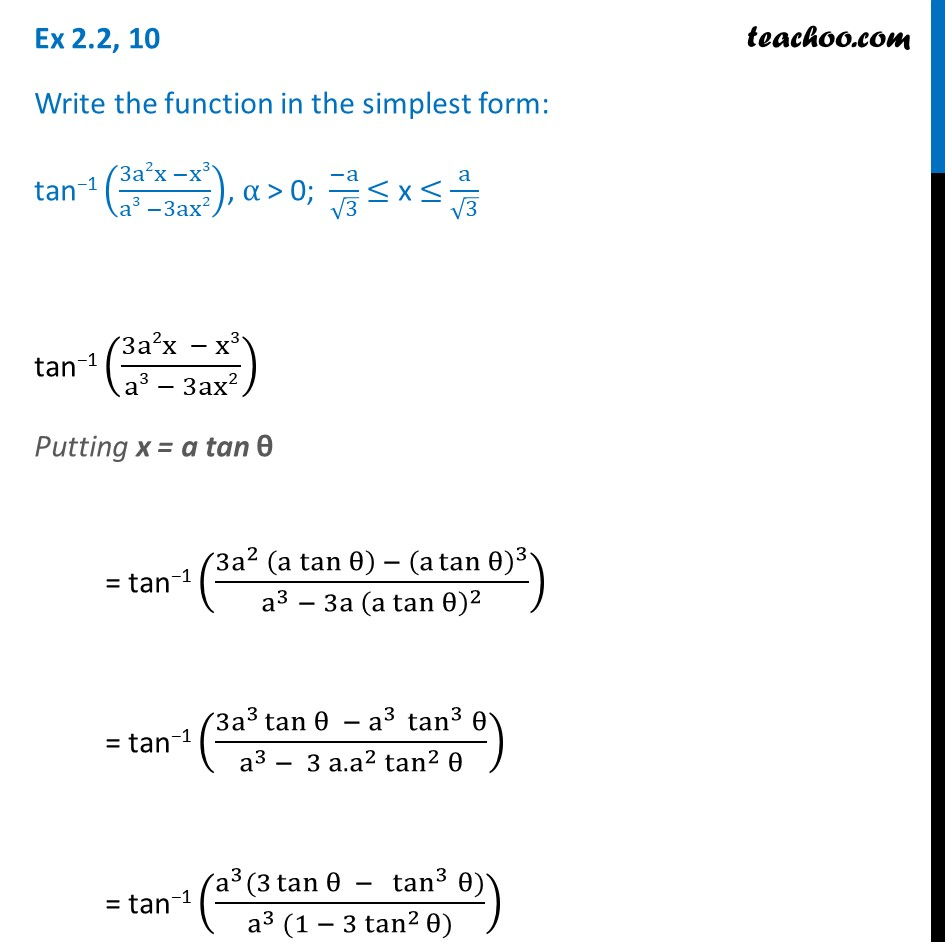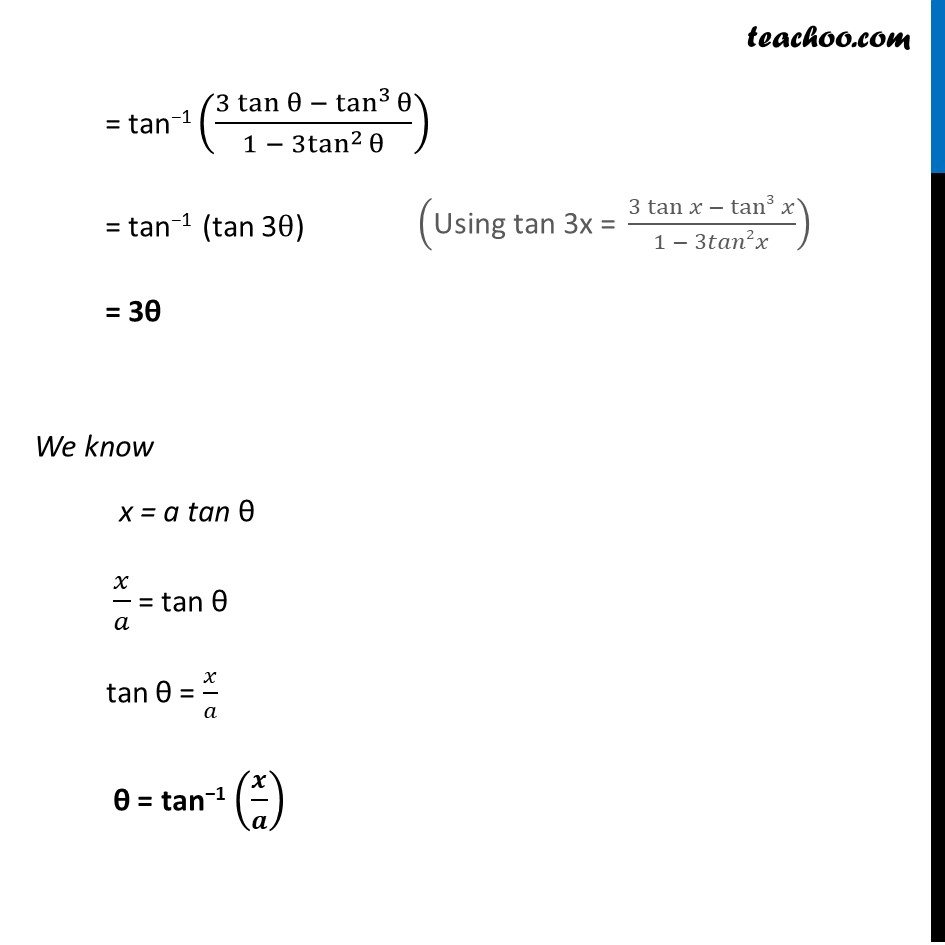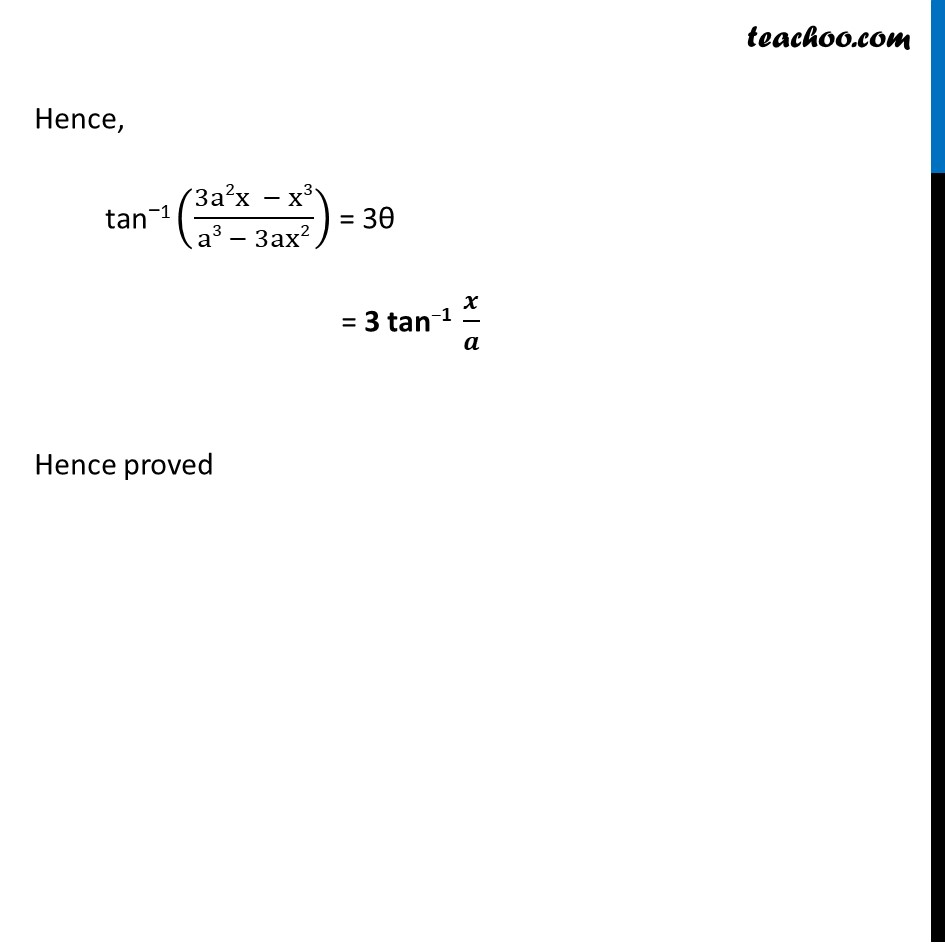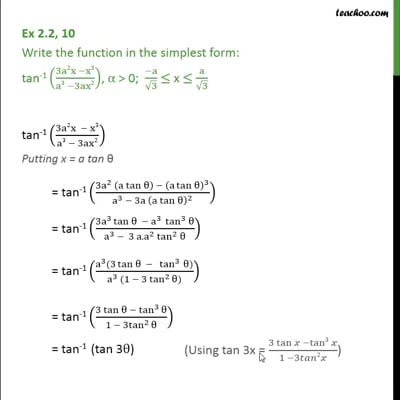Ex 2.2

Chapter 2 Class 12 Inverse Trigonometric Functions
Serial order wiseThis video is only available for Teachoo black users

Maths Crash Course - Live lectures + all videos + Real time Doubt solving!

### Transcript

Ex 2.2, 10 Write the function in the simplest form: tan−1 ((3a2x −x3)/(a3 −3ax2)), α > 0; (−a)/√3 ≤ x ≤ a/√3 tan−1 ((3a2x − x3)/(a3 − 3ax2)) Putting x = a tan θ = tan−1 ((3a^2 (a tan θ) − (a tan θ)^3)/(a^3 − 3a (a tan θ)^2 )) = tan−1 ((3a^3 tan θ − a^3 tan^3 θ)/(a^3 − 3 a.a^2 tan^2 θ)) = tan−1 ((a^3 (3 tan θ − tan^3 θ))/(a^3 (1 − 3 tan^2 θ))) = tan−1 ((3 tan θ − tan^3 θ)/(1 − 3tan^2 θ)) = tan−1 (tan 3θ) = 3θ We know x = a tan θ 𝑥/𝑎 = tan θ tan θ = 𝑥/𝑎 θ = tan−1 (𝒙/𝒂) ("Using tan 3x = " (3 tan⁡〖𝑥 − tan3⁡𝑥 〗)/(1 − 3𝑡𝑎𝑛2𝑥)) Hence, tan−1 ((3a2x − x3)/(a3 − 3ax2)) = 3θ = 3 tan−1 𝒙/𝒂 Hence proved